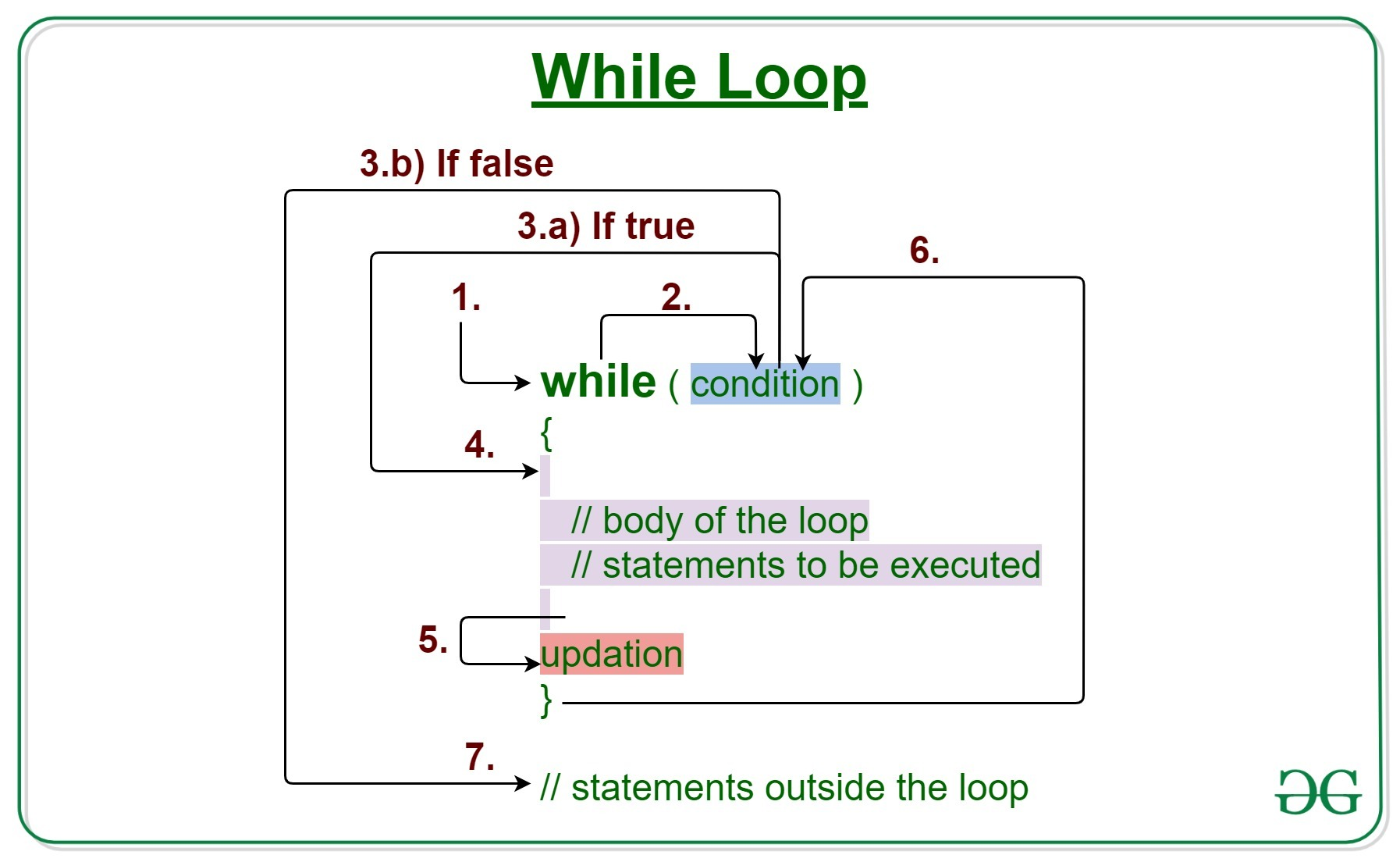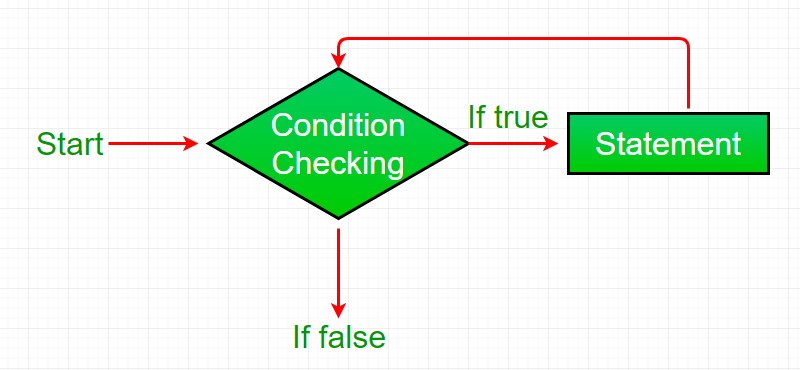Open in App
Not now

# Java while loop with Examples

• Difficulty Level : Easy
• Last Updated : 23 Jun, 2022

Java while loop is a control flow statement that allows code to be executed repeatedly based on a given Boolean condition. The while loop can be thought of as a repeating if statement. While loop in Java comes into use when we need to repeatedly execute a block of statements. The while loop is considered as a repeating if statement. If the number of iterations is not fixed, it is recommended to use the while loop.Syntax:

```while (test_expression)
{
// statements

update_expression;
}```

The various parts of the While loop are:

1. Test Expression: In this expression, we have to test the condition. If the condition evaluates to true then we will execute the body of the loop and go to update expression. Otherwise, we will exit from the while loop.

Example:

`i <= 10`

2. Update Expression: After executing the loop body, this expression increments/decrements the loop variable by some value.

Example:

`i++;`

How Does a While loop execute?

1. Control falls into the while loop.
2. The flow jumps to Condition
3. Condition is tested.
• If Condition yields true, the flow goes into the Body.
• If Condition yields false, the flow goes outside the loop
4. The statements inside the body of the loop get executed.
5. Updation takes place.
6. Control flows back to Step 2.
7. The while loop has ended and the flow has gone outside.

Flowchart For while loop (Control Flow):Example 1: This program will try to print “Hello World” 5 times.

## Java

 `// Java program to illustrate while loop.`   `class` `whileLoopDemo {` `    ``public` `static` `void` `main(String args[])` `    ``{` `        ``// initialization expression` `        ``int` `i = ``1``;`   `        ``// test expression` `        ``while` `(i < ``6``) {` `            ``System.out.println(``"Hello World"``);`   `            ``// update expression` `            ``i++;` `        ``}` `    ``}` `}`

Output

```Hello World
Hello World
Hello World
Hello World
Hello World```

Time Complexity: O(1)

Auxiliary Space : O(1)

Dry-Running Example 1: The program will execute in the following manner.

```1. Program starts.
2. i is initialized with value 1.
3. Condition is checked. 1 < 6 yields true.
3.a) "Hello World" gets printed 1st time.
3.b) Updation is done. Now i = 2.
4. Condition is checked. 2 < 6 yields true.
4.a) "Hello World" gets printed 2nd time.
4.b) Updation is done. Now i = 3.
5. Condition is checked. 3 < 6 yields true.
5.a) "Hello World" gets printed 3rd time
5.b) Updation is done. Now i = 4.
6. Condition is checked. 4 < 6 yields true.
6.a) "Hello World" gets printed 4th time
6.b) Updation is done. Now i = 5.
7. Condition is checked. 5 < 6 yields true.
7.a) "Hello World" gets printed 5th time
7.b) Updation is done. Now i = 6.
8. Condition is checked. 6 < 6 yields false.
9. Flow goes outside the loop. Program terminates.
```

Example 2: This program will find the summation of numbers from 1 to 10.

## Java

 `// Java program to illustrate while loop`   `class` `whileLoopDemo {` `    ``public` `static` `void` `main(String args[])` `    ``{` `        ``int` `x = ``1``, sum = ``0``;`   `        ``// Exit when x becomes greater than 4` `        ``while` `(x <= ``10``) {` `            ``// summing up x` `            ``sum = sum + x;`   `            ``// Increment the value of x for` `            ``// next iteration` `            ``x++;` `        ``}` `        ``System.out.println(``"Summation: "` `+ sum);` `    ``}` `}`

Output

`Summation: 55`

Time Complexity: O(1)

Auxiliary Space : O(1)

Related Articles:

My Personal Notes arrow_drop_up
Related Articles